## Question Help In a study of cell phone usage and brain hemisphen dominance, an Internet survey was e-mailed to 6957 subjects randomly

Question

Question Help
In a study of cell phone usage and brain hemisphen dominance, an Internet survey was e-mailed to 6957 subjects randomly selected from an online group involved with ears. There were 1319 surveys returned Use a 0.01
significance level to test the claim that the return rate is less than 20% Use the P-value method and use the normal distnbution as an approximation to the binomial distrbution
Identify the null hypothesis and alternative hypothesis

in progress 0
5 months 2021-09-05T09:10:37+00:00 1 Answers 5 views 0

The null hypothesis is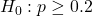The alternative hypothesis is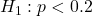The p-value of the test is of 0.0154 > 0.01, which means that at the 0.01 significance level, there is not significant evidence that the return rate is less than 20%.

Step-by-step explanation:

Test the claim that the return rate is less than 20%.

At the null hypothesis, we test if the return rate is of at least 20%, that is:At the alternative hypothesis, we test if the return rate is less than 20%, that is:Normal distribution as an approximation to the binomial distribution to find the Z-score.

Binomial probability distribution

Probability of exactly x successes on n repeated trials, with p probability.

Can be approximated to a normal distribution, using the expected value and the standard deviation.

The expected value of the binomial distribution is: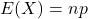The standard deviation of the binomial distribution is: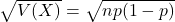Normal probability distribution

Problems of normal distributions can be solved using the z-score formula.

In a set with mean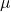and standard deviation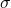, the z-score of a measure X is given by: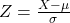The Z-score measures how many standard deviations the measure is from the mean. After finding the Z-score, we look at the z-score table and find the p-value associated with this z-score. This p-value is the probability that the value of the measure is smaller than X, that is, the percentile of X. Subtracting 1 by the p-value, we get the probability that the value of the measure is greater than X.

When we are approximating a binomial distribution to a normal one, we have that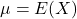,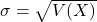.

0.2 is tested at the null hypothesis. Sample of 6957.

This means that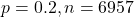. So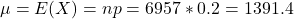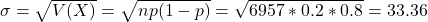1319 surveys returned

This means that, due to continuity correction,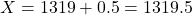. So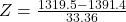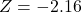P-value of the test:

The p-value of the test is the probability of 1319 or less people returning the survey, which is the p-value of Z = -2.16.

Looking at the z-table, Z = -2.16 has a p-value of 0.0154.

The p-value of the test is of 0.0154 > 0.01, which means that at the 0.01 significance level, there is not significant evidence that the return rate is less than 20%.